# RD Sharma Solutions for Class 9 Maths Chapter 12 Heron's Formula Exercise 12.1

### RD Sharma Class 9 Solutions Chapter 12 Ex 12.1 Free Download

RD Sharma Solutions for Class 9 Maths are given here for Chapter 12 exercise 12.1. In this exercise, students will learn how to find the area of a triangle using heron’s formula with the help of various solved examples.

Heron’s Formula: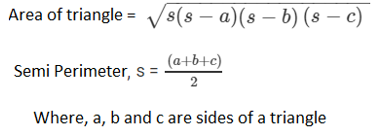The Solutions for RD Sharma Maths chapter 12 exercise 12.1 includes solved problems and step by step explanations given by our experts to further helps students find alternative ways to solve a problem.

## Download PDF of RD Sharma Solutions for Class 9 Maths Chapter 12 Heron’s Formula Exercise 12.1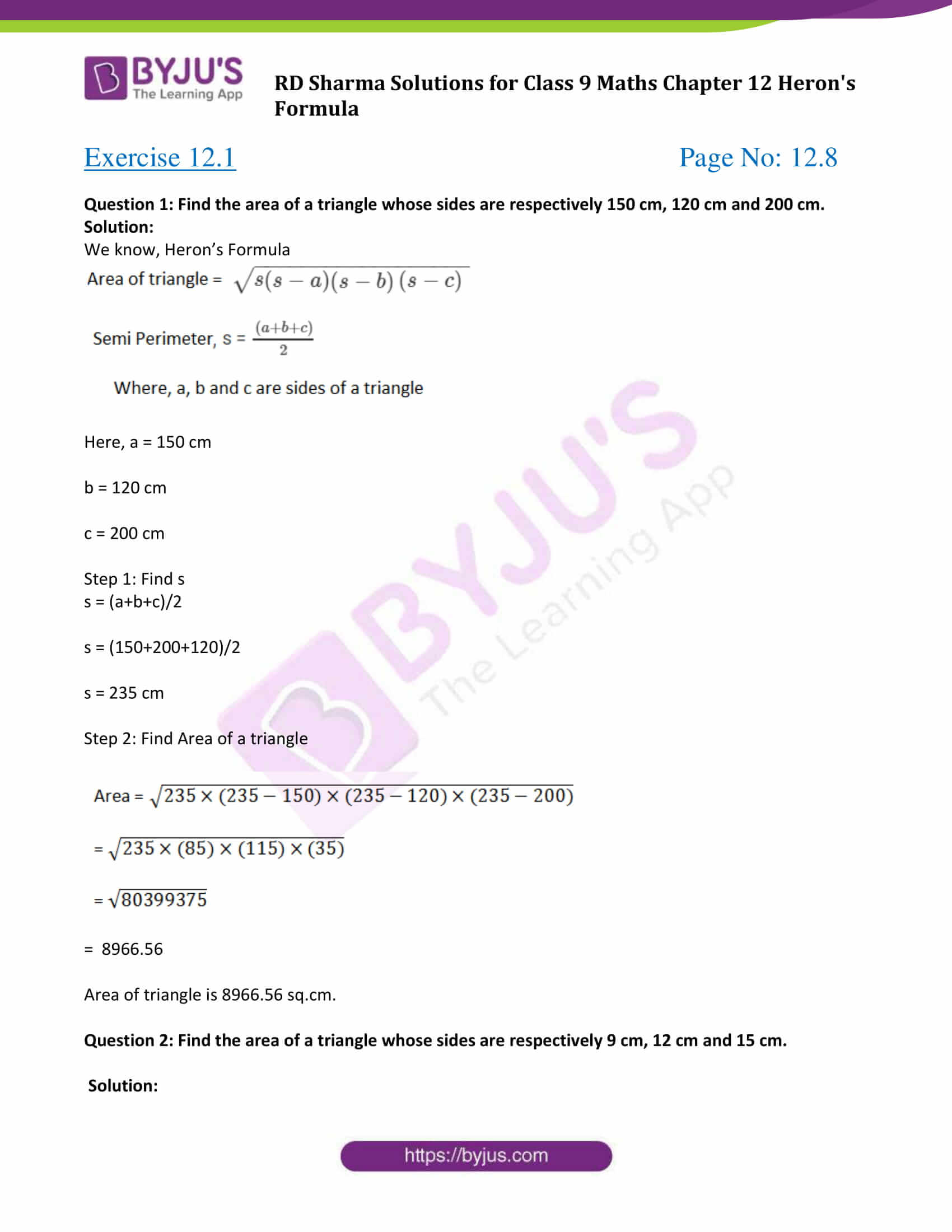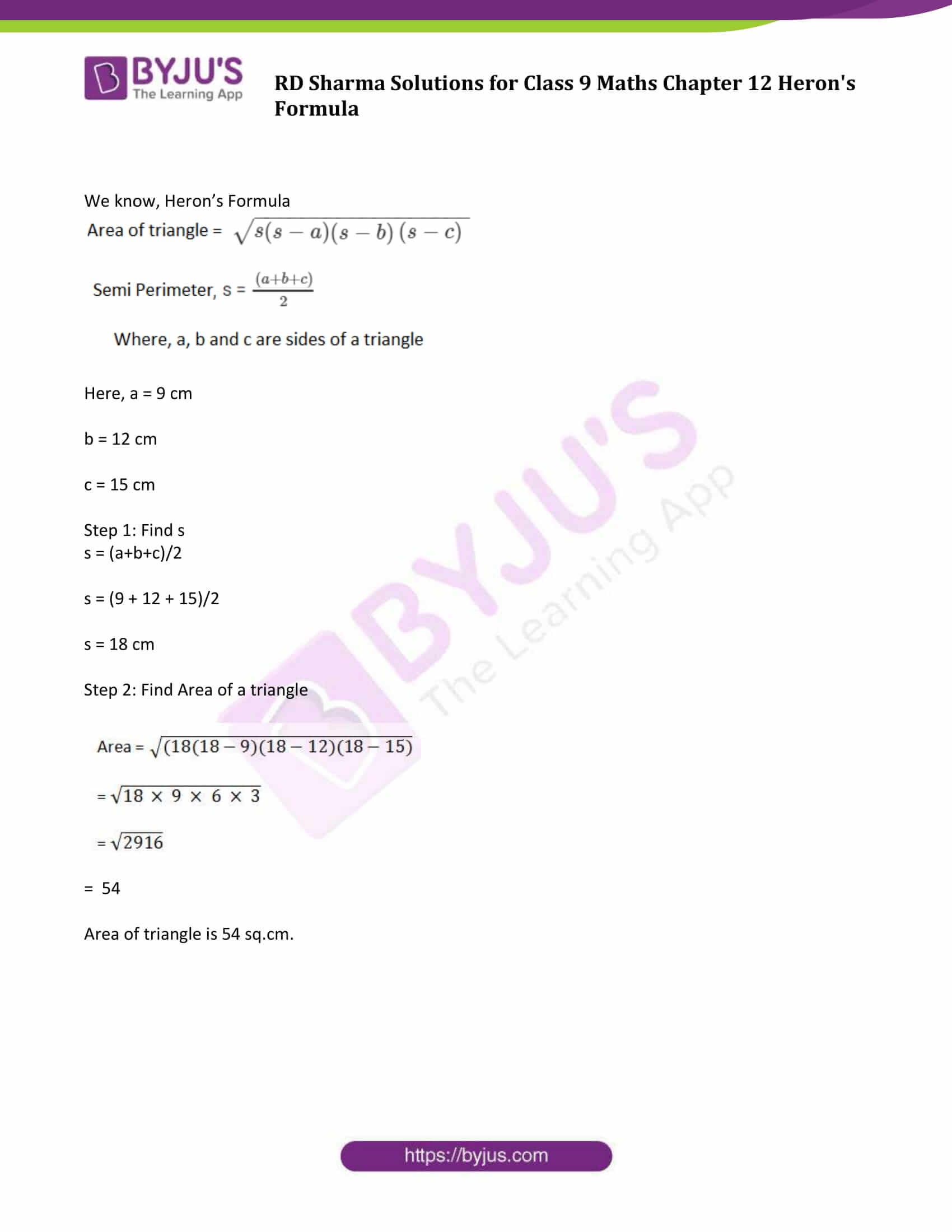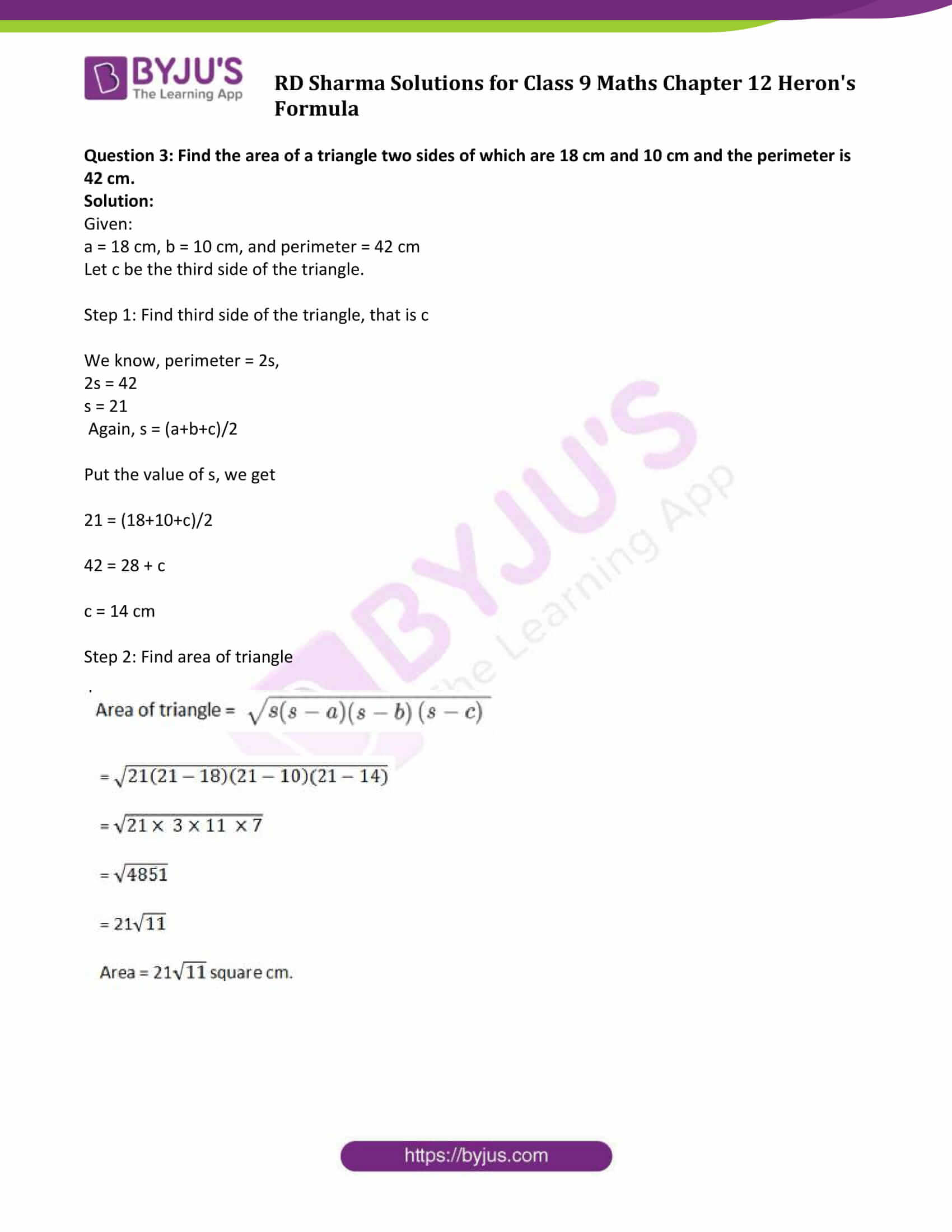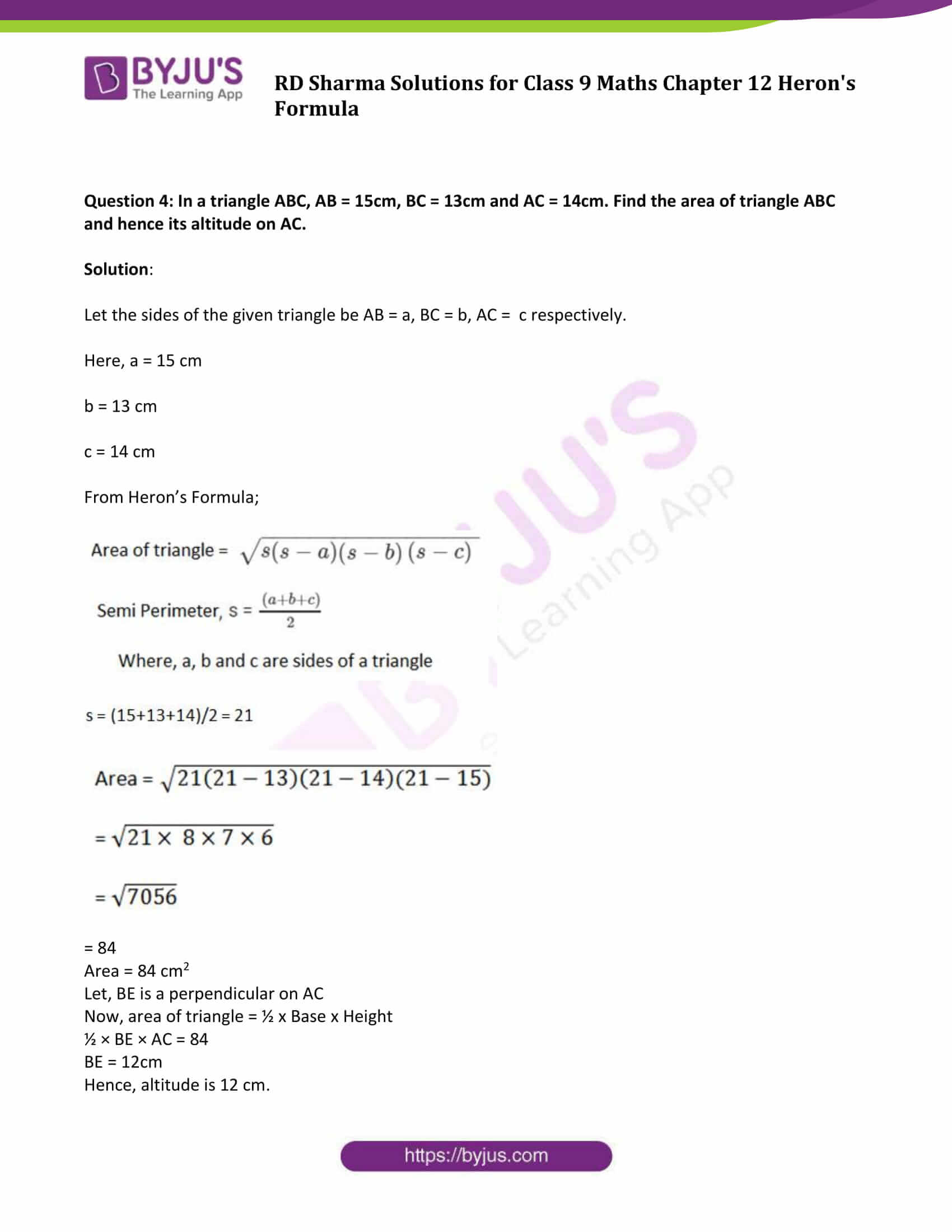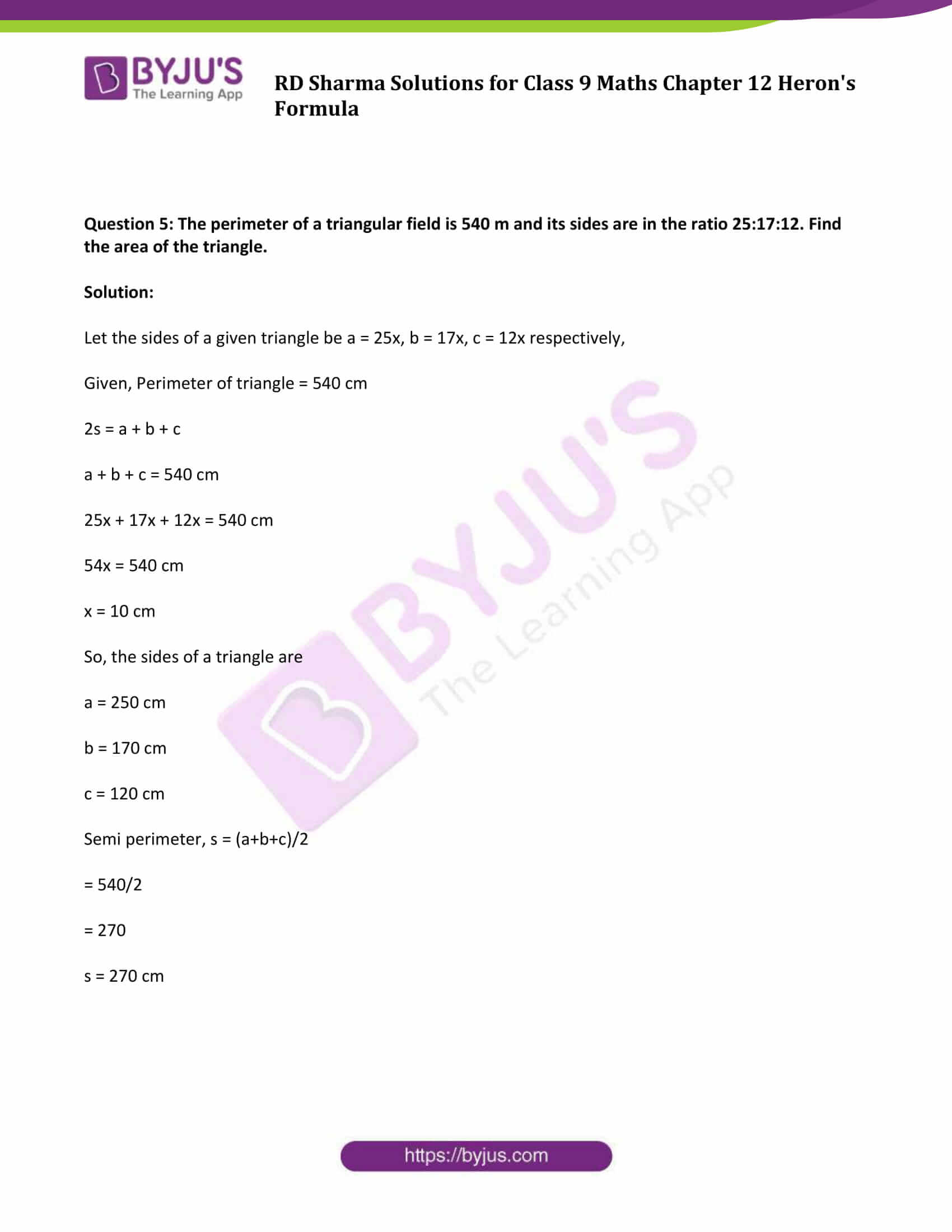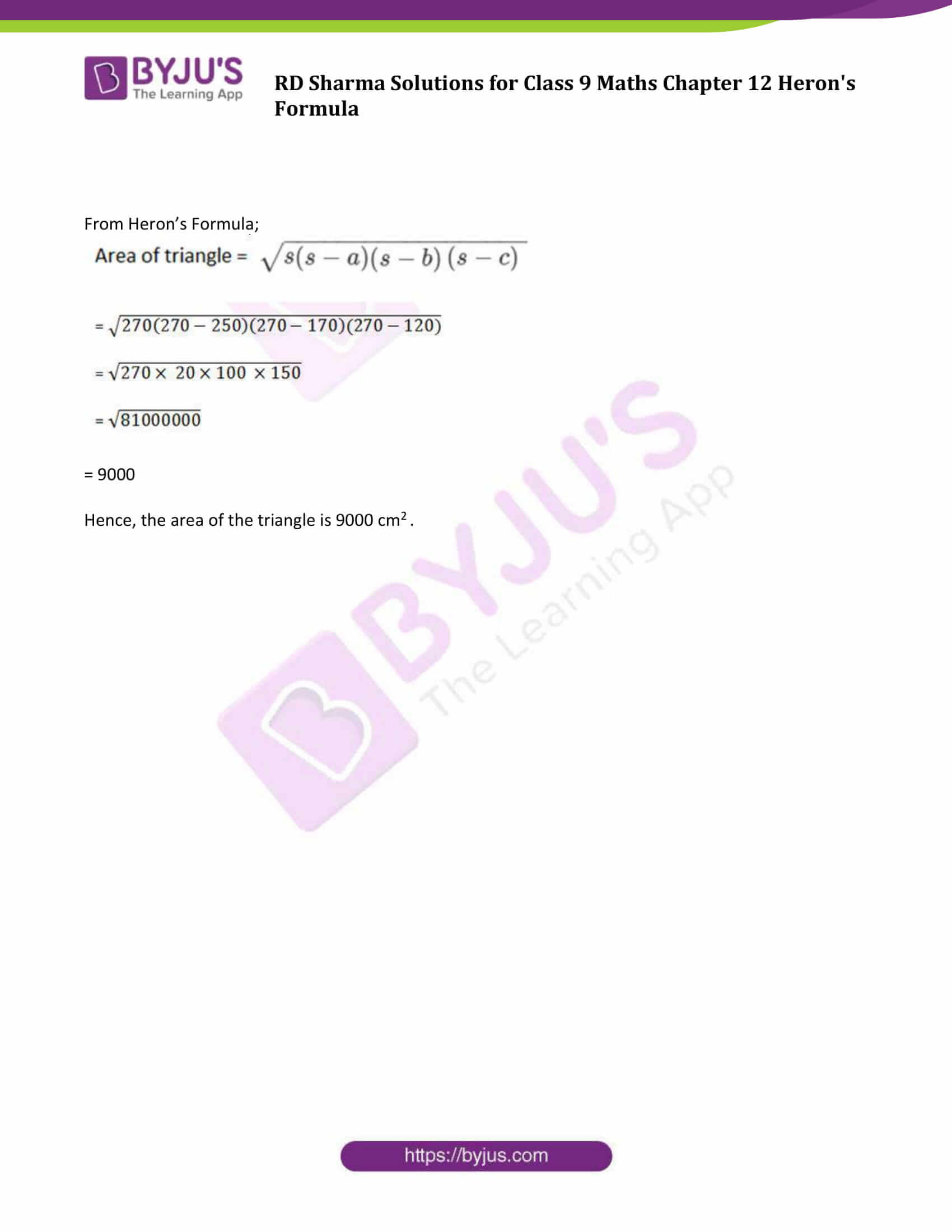## Exercise 12.1 Page No: 12.8

Question 1: Find the area of a triangle whose sides are respectively 150 cm, 120 cm and 200 cm.

Solution:

We know, Heron’s Formula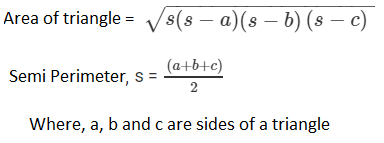Here, a = 150 cm

b = 120 cm

c = 200 cm

Step 1: Find s

s = (a+b+c)/2

s = (150+200+120)/2

s = 235 cm

Step 2: Find Area of a triangle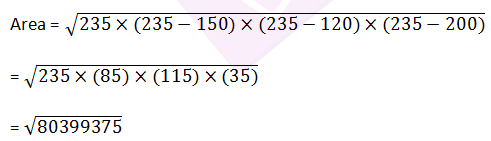= 8966.56

Area of triangle is 8966.56 sq.cm.

Question 2: Find the area of a triangle whose sides are respectively 9 cm, 12 cm and 15 cm.

Solution:

We know, Heron’s FormulaHere, a = 9 cm

b = 12 cm

c = 15 cm

Step 1: Find s

s = (a+b+c)/2

s = (9 + 12 + 15)/2

s = 18 cm

Step 2: Find Area of a triangle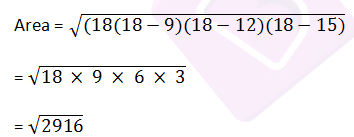= 54

Area of triangle is 54 sq.cm.

Question 3: Find the area of a triangle two sides of which are 18 cm and 10 cm and the perimeter is 42 cm.

Solution:

Given:

a = 18 cm, b = 10 cm, and perimeter = 42 cm

Let c be the third side of the triangle.

Step 1: Find third side of the triangle, that is c

We know, perimeter = 2s,

2s = 42

s = 21

Again, s = (a+b+c)/2

Put the value of s, we get

21 = (18+10+c)/2

42 = 28 + c

c = 14 cm

Step 2: Find area of triangle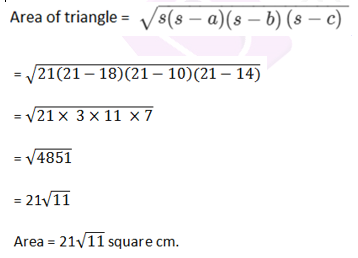Question 4: In a triangle ABC, AB = 15cm, BC = 13cm and AC = 14cm. Find the area of triangle ABC and hence its altitude on AC.

Solution:

Let the sides of the given triangle be AB = a, BC = b, AC = c respectively.

Here, a = 15 cm

b = 13 cm

c = 14 cm

From Heron’s Formula;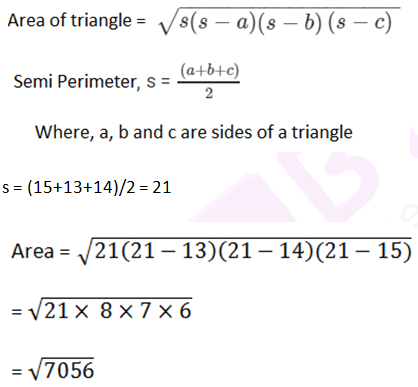= 84

Area = 84 cm2

Let, BE is a perpendicular on AC

Now, area of triangle = ½ x Base x Height

½ × BE × AC = 84

BE = 12cm

Hence, altitude is 12 cm.

Question 5: The perimeter of a triangular field is 540 m and its sides are in the ratio 25:17:12. Find the area of the triangle.

Solution:

Let the sides of a given triangle be a = 25x, b = 17x, c = 12x respectively,

Given, Perimeter of triangle = 540 cm

2s = a + b + c

a + b + c = 540 cm

25x + 17x + 12x = 540 cm

54x = 540 cm

x = 10 cm

So, the sides of a triangle are

a = 250 cm

b = 170 cm

c = 120 cm

Semi perimeter, s = (a+b+c)/2

= 540/2

= 270

s = 270 cm

From Heron’s Formula;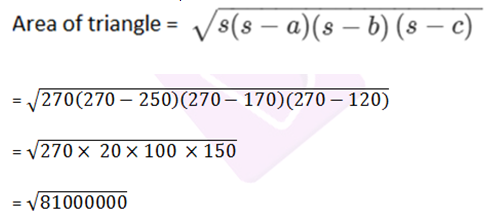= 9000

Hence, the area of the triangle is 9000 cm2 .

## RD Sharma Solutions for Class 9 Maths Chapter 12 Exercise 12.1

Class 9 Maths Chapter 12 Heron’s Formula Exercise 12.1 is based on Heron’s formula. Find detailed RD Sharma solutions for class 9 Maths chapter 12, solved by BYJU’S experts using step-by-step problem solving method here. Students can freely download RD Sharma solutions and sharpen their skills.